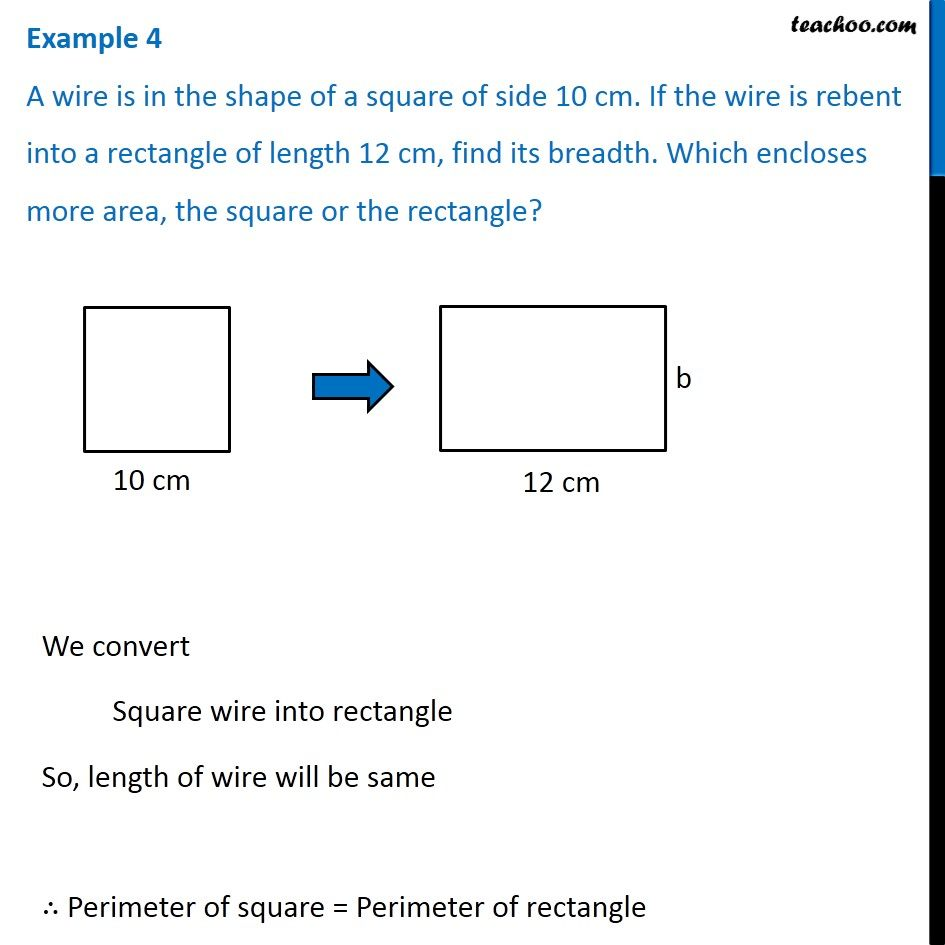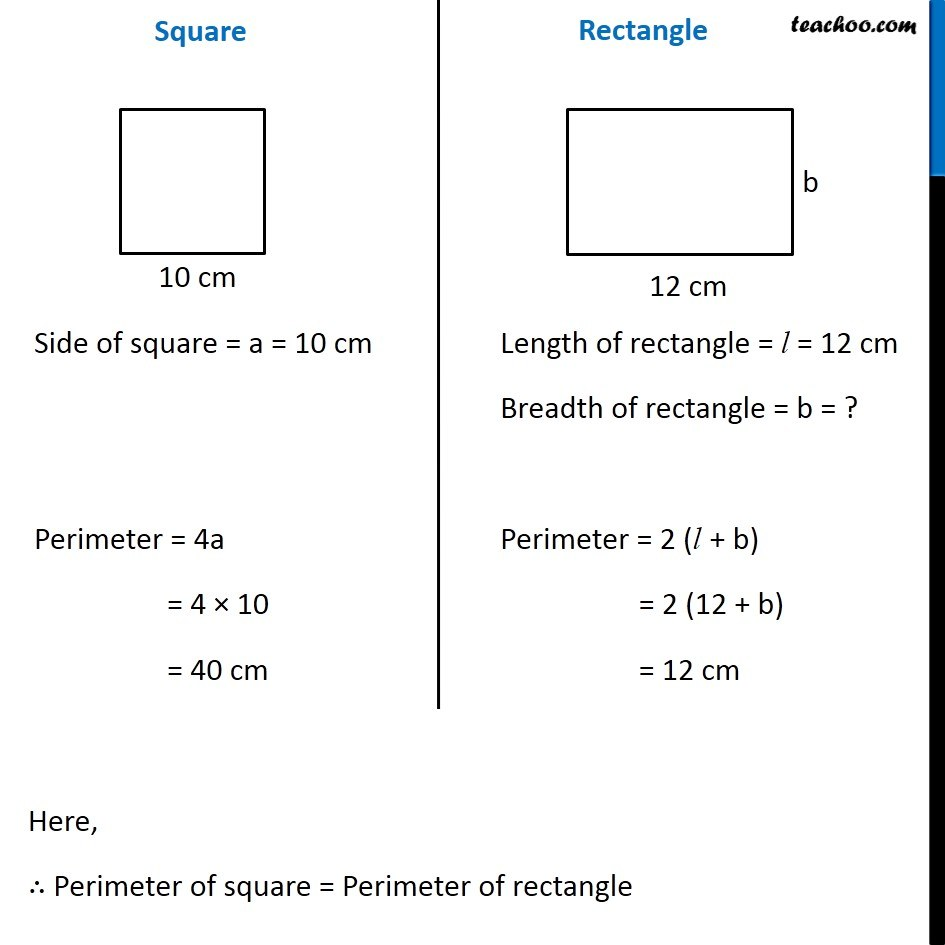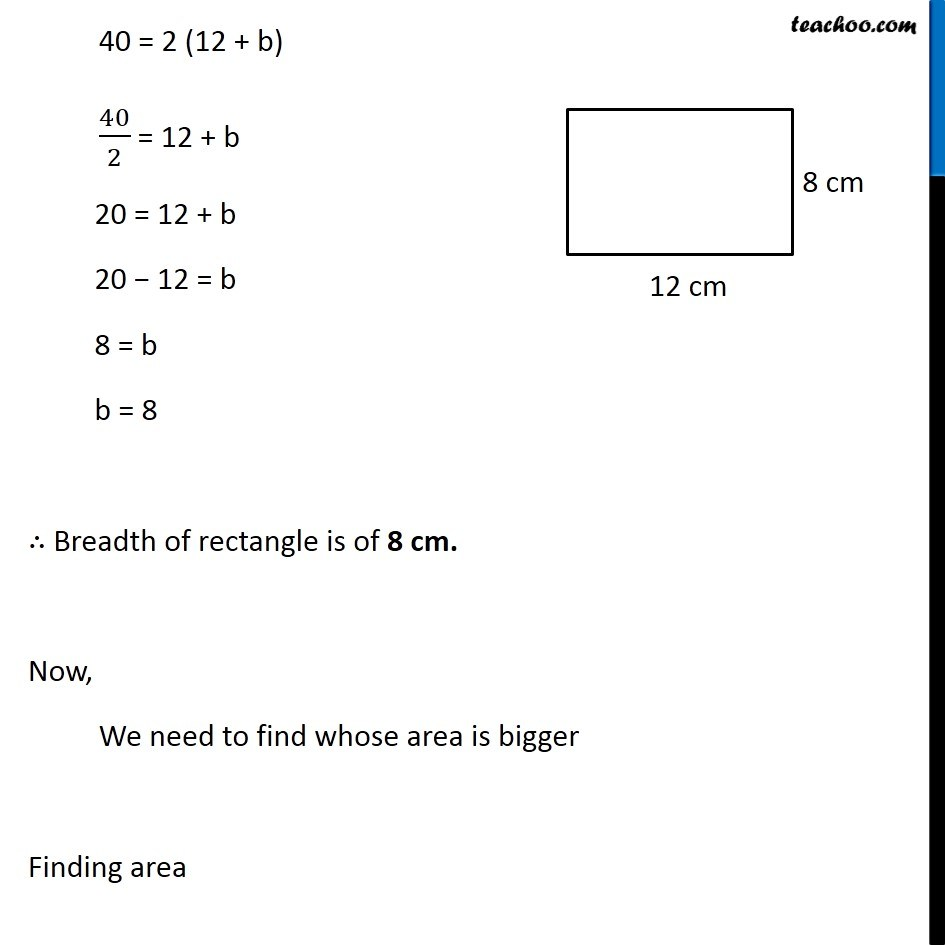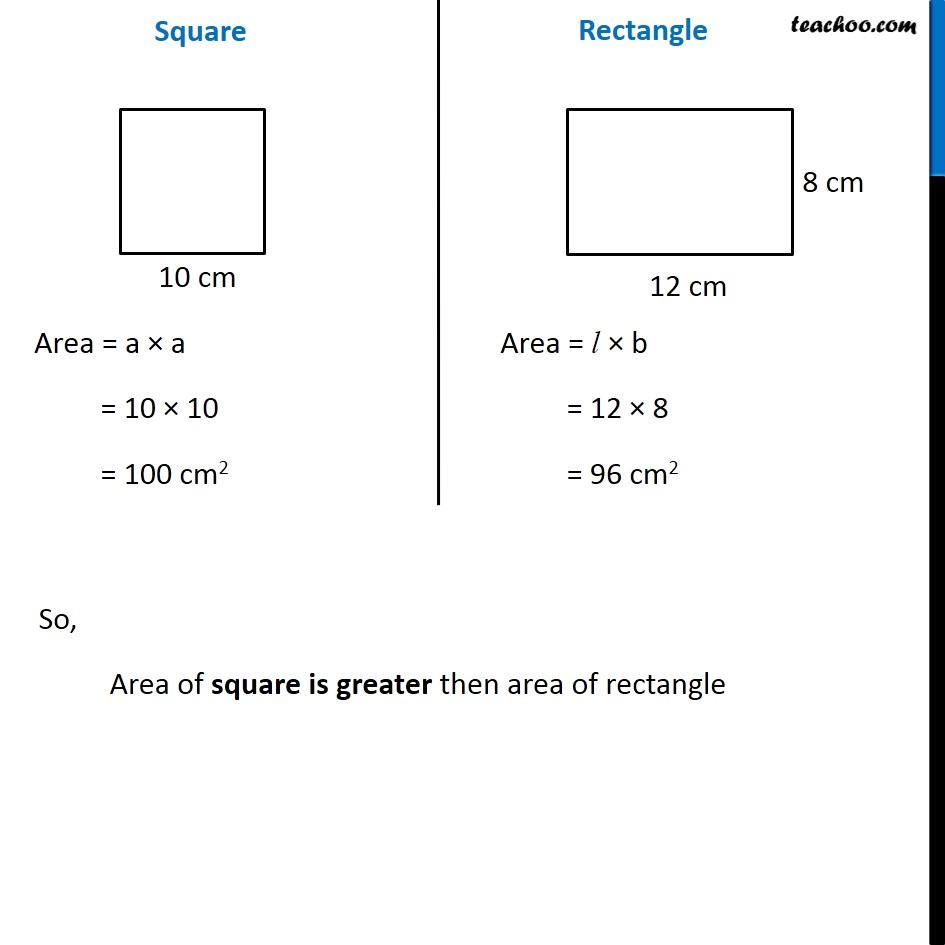Converting Square to Rectangle

Chapter 9 Class 7 Perimeter and Area
Concept wiseLearn in your speed, with individual attention - Teachoo Maths 1-on-1 Class

### Transcript

Question 4 A wire is in the shape of a square of side 10 cm. If the wire is rebent into a rectangle of length 12 cm, find its breadth. Which encloses more area, the square or the rectangle? We convert Square wire into rectangle So, length of wire will be same ∴ Perimeter of square = Perimeter of rectangle Square Side of square = a = 10 cm Perimeter = 4a = 4 × 10 = 40 cm Rectangle Length of rectangle = l = 12 cm Breadth of rectangle = b = ? Perimeter = 2 (l + b) = 2 (12 + b) = 12 cm Here, ∴ Perimeter of square = Perimeter of rectangle 40 = 2 (12 + b) 40/2 = 12 + b 20 = 12 + b 20 − 12 = b 8 = b b = 8 ∴ Breadth of rectangle is of 8 cm. Now, We need to find whose area is bigger Finding area Square Area = a × a = 10 × 10 = 100 cm2 Rectangle Area = l × b = 12 × 8 = 96 cm2 So, Area of square is greater then area of rectangle